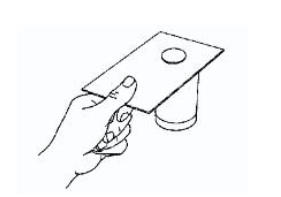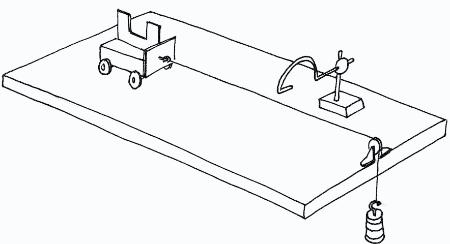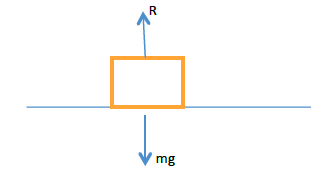The Concept of Inertia
Explain the concept of inertia
Inertia is the ability of a resting body to resist motion or a moving body to continue moving in a straight line when abruptly stopped.
The more mass a body has, the greater its inertia and vice versa is true.
Newton's First Law of Motion
State Newton's first law of Motion
Newton’s 1st law of motion states that “Everybody will continue in its state of rest or of uniform motion unless an external force acts upon it”
Verification of Newton's First Law of Motion
Verify Newton's first law of Motion
Activity 1
Experiment
Aim: To verify Newton’s 1st law of motion.
Materials and apparatus: Glass, manila card and small coin.
Procedures.
A small coin is placed on a manila card and the card is positioned on top of the glass such that the coin is directly positioned over the open mouth of the bottle.Flick the card at C. Make sure that the card is not tilted by moving the finger in the horizontal plane.
Observation: When the card is flicked away quickly by finger, the coin drops neatly into the glass. The coin dropped into the glass because there was no force applied on it when the card was flipped.
Conclusion: The coin continued to be at rest as the card was flicked quickly. This experiment verify Newton’s 1st law of motion.
The Concept of Linear Momentum
Explain concept of linear momentum
Linear momentum of a body is the product of mass and velocity of that body.
Momentum = Mass, m x Velocity, v
Hence P = mv
The SI Unit of Linear Momentum
State the SI unit of linear momentum
The unit of momentum is kilogram meter per second(kgm/s)
Linear Momentum
Determine linear momentum
When two bodies, a heavy one and the light one are acted upon by an external force at the same time(collide) the light body builds up a higher velocity than the heavy one but the momentum they gain remain the same in both cases.
i.e Momentum before collision = Momentum after collision. This is what we call the conservation of momentum and is described by Newton’s 2nd law of motion.
Newton's Second Law of Motion
State Newton's second law of Motion
Newton’s 2nd law of motion states that “The rate of change of momentum is proportional to the applied force and it takes place in the direction of a force”
Consider a body of mass, (m) acted by an external force (f) from an initial velocity (u) to the final velocity (v) within a time interval (t).
Change of momentum = mv – muHence the Newton’s 2nd law of motion can be summarized as;
“The force is directly proportional to acceleration of the object and the acceleration of the same body is inversely proportional to its mass”
F α ma
F = kma but k = 1
Hence F = ma
If a mass of 1kg is accelerated with an acceleration of 1m/sÇ then the force of 1N is said to be acting on it.
Newton is the force which when acting on a body of mass 1kg it produces an acceleration of 1m/s..
Verification of Newton's Second Law of Motion
Verify Newton's second law of Motion
A trolley experiences an acceleration when an external force is applied to it. The aim of this datalogging experiment is explore the relationship between the magnitudes of the external force and the resulting acceleration.
Apparatus and materials
• Light gate, interface and computer
• Dynamics trolley
• Pulley and string
• Slotted masses, 400 g
• Mass, 1 g
• Clamp
• Ruler
• Double segment black card (see diagram)
Take care when masses fall to the floor. Use a box or tray lined with bubble wrap (or similar) under heavy objects being lifted. This will prevent toes or fingers from being in the danger zone.Procedure
1. Select the falling mass to be 100 g. Pull the trolley back so that the mass is raised to just below the pulley. Position the light gate so that it will detect the motion of the trolley soon after it has started moving.Set the software to record data, then release the trolley. Observe the measurement for the acceleration of the trolley.
2. Repeat this measurement from the same starting position for the trolley several times. Enter from the keyboard '1'( 1 newton) in the force column of thetable.
3. Transfer 100 g from the trolley to the slotted mass, to increase it to 200 g. Release the trolley from the same starting point as before. Repeat this several times. Enter '2' (2 newtons) in the force column of the table.
4. Repeat the above procedure for slotted masses of 300 g and 400 g.
Difference between Elastic and Inelastic Collisions
Distinguish between Elastic and Inelastic Collisions
Elastic collision
This is the type of collision whereby each body moves with a separate velocity after collision. In this type of collision both energy and momentum are conserved.Inelastic collision
Is the type of collision whereby all bodies move with the same velocity after collision. This velocity is known as common velocity. In this type of collision energy is not conserved, only momentum is conserved.Impulse is the change of momentum which is given asm the product of force and the time taken to change momentum.F = mv – mu –Ft is the impulse of a force which is given by mv – mu.
The Principle of Conservation of Linear Momentum
State the principle of conservation of linear Momentum
Principle of conservation of linear momentum states that, “When two or more bodies acts upon one another; that is when they collide their total momentum remains constant, provided that there is no external force acting”
Momentum before collision = Momentum after collision
Consider two bodies of masses m₁ and m₂ moving with initial velocities u₁ and u₂ and then move with final velocities v₁ and v₂ respectively after they collide one another.
From the principle of conservation of momentum: Momentum before collision = Momentum after collision
m₁u₁ + m₂u₂ = m₁v₁ + m₂v₂
The Principle of Conservation of Linear Momentum in Solving Problems
Apply the principle of conservation of linear momentum in solving problems
Activity 2
Apply the principle of conservation of linear momentum in solving problems
Difference between Action and Reaction Forces
Distinguish between Action and Reaction Forces
Consider a book of mass, m which is at rest on a table. This book will exert on a table with a force equal to its weight. The table exert an equal upward force.
The downward force exerted by the book(weight) on the table is known as action force while the upward force exerted by the table on the book is known as reaction force.
These two forces acts in opposite direction but they are equal in magnitude.Where; R = reaction, mg=weight of a book
Newton's Third Law of Motion
State Newton's third Law of Motion
Newton’s 3rdlaw of motionstates that “To every action there is an equal and opposite reaction”
Application of Newton's Third Law of Motion
Apply Newton's third Law of Motion
The person firing a gun will feel the recoil when the bullet leaves the gun.
Consider a gun of mass mg ejects a bullet of mass mb with a velocity vb and the gun recoils with velocity vg.
From the principle of conservation of momentum:
Recoil momentum of gun = Momentum of bullet
GET NOTES THROUGH WHATSAPP/EMAIL BY PAYING LITTLE AMOUNT OF MONEY, CONTACT US 0759146185/ 0622105865